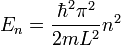# Infinite potential well

omri3012
infinite potential well and the uncertainty principle

the solution for Schroedinger equation in infinite potential well satisfy the following

energy levels:where l is the width of the well.

E can't be zero since then $$\psi$$=0 so there isn't any particle in the well . i read in

a book that "there is a tight connection between this fact (E$$\neq$$0) and the

uncertainty principle", what exactly is the connection?

Last edited:

## Answers and Replies

neworder1
I don't know what the author meant, but my guess would be:
- since the well has finite width, the uncertainty in position is always finite, i.e. $$\Delta X < \infty$$
- now, if you take time-independent Schroedinger equation $$H\Psi=E\Psi \Leftrightarrow \frac{\partial^2 \Psi}{\partial x^2}=\frac{-2mE}{\hbar^2}\Psi$$ and put this into momentum uncertainty: $$\langle P^2 \rangle - \langle P \rangle^2$$ and calculate the integrals, you obtain that $$(\Delta P)^2$$ is something like $$\frac{-2mE}{\hbar^2}$$. So $$E=0$$ would violate uncertainty principle, since for $$E=0$$ $$\Delta P = 0$$, $$\Delta X < \infty$$

Last edited:
omri3012
Thanks for your time, i really appreciate it.

but i didn't understand two things:

1. how can you assume that $$H\Psi=E\Psi$$?

2. why does $$\Delta X < \infty$$ imply for contradiction in the uncertainty principle?

neworder1
Ad. 1. When trying to determine possible energy values, we look first for a separated solution to the Schrodinger equation, i.e. solution of the form $$\psi (x, t) = \Psi (x) \phi (t)$$. If the Hamiltonian $$H$$ is time-independent, separation of variables proves that $$H\Psi = E\Psi$$ for some constant $$E$$ (look this part up in any textbook). Now we have to determine possible values of $$E$$, and the argument above show that we cannot have $$E=0$$. This is actually a bit of an overkill, since the equation $$\frac{\partial^2 \Psi}{\partial x^2}=0$$, along with boundary conditions $$\Psi (0)=\Psi(L)=0$$, gives $$\Psi = 0$$ instantly, but I guess this is what the author had in mind.

Ad. 2. The uncertainty principle says that $$\Delta X \Delta P \geq \frac{\hbar}{2}$$. For $$E=0$$ we get $$\Delta P=0$$ as well, so the uncertainty principle could be satisfied only if $$\Delta X = \infty$$. But the well has finite width, so position uncertainty is also finite.

Obviously, this is a very roundabout way of proving that $$E \neq 0$$.

omri3012
Thanks,

now it's all clear